# Inductive sensing: Sensor frequency constraints

Configuration of inductance-to-digital converter (LDC) sensors can seem quite challenging, but by using the graph introduced in this blog post, the process can be greatly simplified.

For LDCs, a sensor is a simple inductor, spring, or PCB coil in parallel with a capacitor. One of the primary constraints on the sensor is the operating frequency. So, we start with the equation which describes the oscillation frequency of a sensor: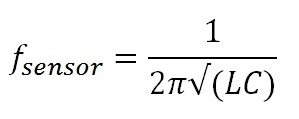Where:

• fSENSOR is the sensor oscillation frequency,
• L is the inductance of the sensor, and
• C is the parallel capacitance.

Using the example of the LDC1000, which has a sensor oscillation frequency range of 5 kHz < fsensor <5 MHz, we can draw the following graph:Figure 1: Graph of constant frequency lines

As an example, if we have a sensor coil with an inductance of 100 µH and want an oscillation frequency of 600 kHz, we need to use a 704 pF capacitor. If we wanted an oscillation frequency of 5 MHz, we would need to use a 10.1 pF capacitor (Figure 2.)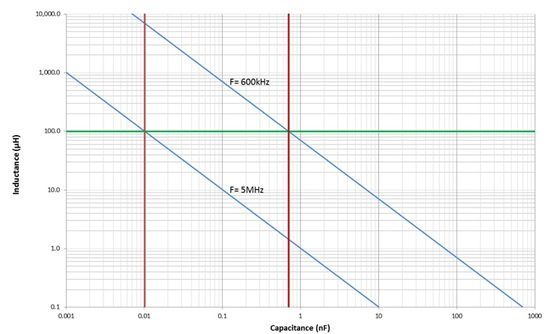Figure 2: 5 MHz and 600 kHz sensors

However, there are constraints on selecting the capacitor value beyond choosing a sensor oscillation frequency. One constraint is parasitic capacitances - they are typically not very stable, and it is very easy to get a few pF of parasitic capacitance in many physical systems. This can have a significant impact on the measured inductance. If our sensor is 100 µH in parallel with 10.1 pF, and we have just 0.5 pF of parasitic capacitance, the measurement frequency would change from 5 MHz to 4.88 MHz. This is equivalent to a shift in inductance of 5 µH!

If we change the capacitor to 704 pF, our sensor frequency is now 600 kHz, but the same parasitic shift of 0.5 pF would cause only a shift from 600 Hz to 599.8 kHz, which would appear to be a change in inductance of only 0.07 µH.

Given these considerations, we add a bound on the lower values of capacitance, and so our graph now looks like: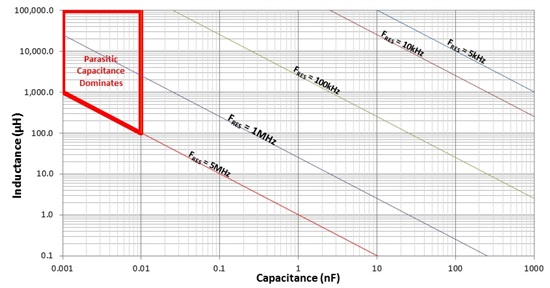Figure 3: Graph with parasitic cap region

In LDC applications, we recommend using C0G-grade capacitors (they are also referred to as NP0 capacitors). These capacitors have many very good characteristics: they are very stable and are available in small footprints for relatively low cost. They also offer minimal aging shifts, good temperature stability, wide temperature operating ranges, low ESR, small dC/dV and excellent distortion.

However, they do not have a large amount of capacitance per unit volume, so it becomes difficult to find C0G capacitors of more than 0.47 µF (and those have a footprint of 5 mm × 5.7 mm!).

These considerations introduce an upper bound on capacitor values, as shown in Figure 4.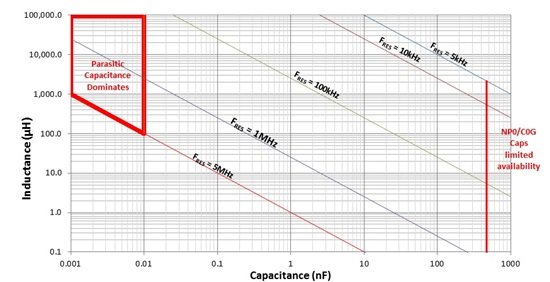Figure 4: Maximum available capacitors

Finally, we need to consider the RP of the sensor. Devices like the LDC1000, LDC1041, and LDC1051 can drive sensors with RP as low as 798 Ω.

The value of RP can be calculated from the Q of the sensor using the equation:Let us pick two sensors, one with Q=5 and the second with Q=25. When we graph the two sensors across the 5 kHz to 5 MHz range, we can add the two lines that correspond to the LDC1000’s minimum RP of 798 Ω. Notice that the higher Q sensor has a larger range of acceptable capacitor and inductance values (Figure 5.)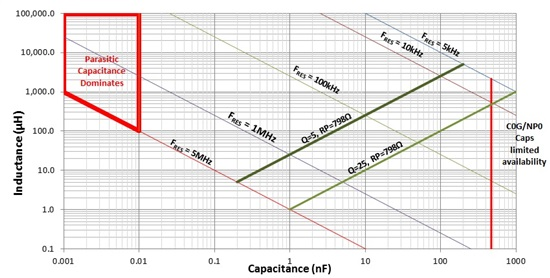Figure 5: RP limitations

With some color-coding, we get the map below: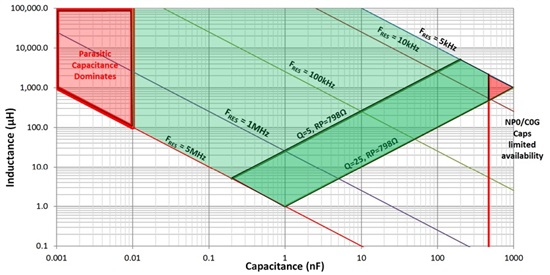Figure 6: Operation region

The light green shaded region is the operational region for a sensor with a Q of 5, while a sensor with a Q of 25 can operate into an extended region, indicated by the dark green shaded region.

Finally, we can see the operational region available. The following constraints need to be followed:

1. While the sensor capacitor can be as small as 10 pF, 100 pF or larger is recommended for most applications.
2. Obtaining sensor capacitors larger than 470 nF can be difficult, so there is a limit on the lowest frequency sensors.
3. While the LDC1000 can operate with sensor inductances as low as 1 µH, a practical minimum sensor inductance is  ~5 µH due to the sensor RP.
4. A higher Q sensor offers more flexibility in selecting a sensor configuration